# Samacheer Kalvi 9th Maths Solutions Chapter 3 Algebra Ex 3.1

## Tamilnadu Samacheer Kalvi 9th Maths Solutions Chapter 3 Algebra Ex 3.1

9th Maths Algebra Exercise 3.1 Question 1.
Which of the following expressions are polynomials. If not give reason:
(i) $$\frac{1}{x^{2}}$$ + 3x – 4
(ii) x2 (x – 1)
(iii) $$\frac{1}{x}$$ (x + 5)
(iv) $$\frac{1}{x^{-2}}+\frac{1}{x^{-1}}$$ + 7
(v) $$\sqrt{5} x^{2}+\sqrt{3} x+\sqrt{2}$$
(vi) $$m^{2}-\sqrt{m}+7 m-10$$
Solution: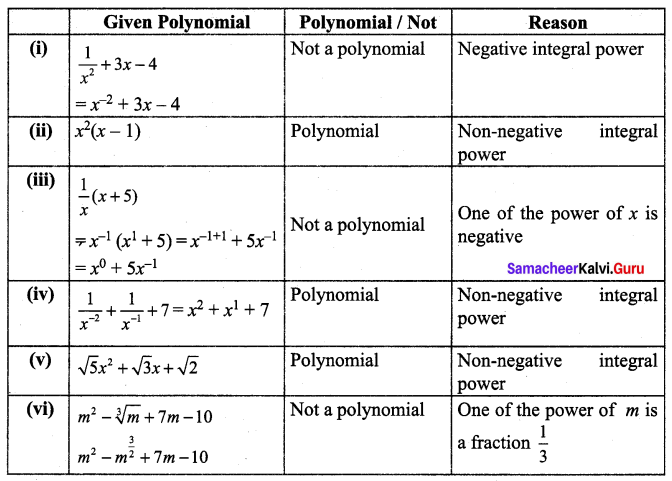9th Maths Exercise 3.1 Question 2.
Write the coefficient of x2 and x in each of the following polynomials,
(i) 4 + $$\frac{2}{5} x^{2}$$ – 3x
(ii) 6 – 2x2 + 3x3 – $$\sqrt{7} x$$
(iii) πx2 – x + 2
(iv) $$\sqrt{3} x^{2}+\sqrt{2} x$$ + 0.5
(v) x2 – $$\frac{7}{2} x$$ + 8
Solution: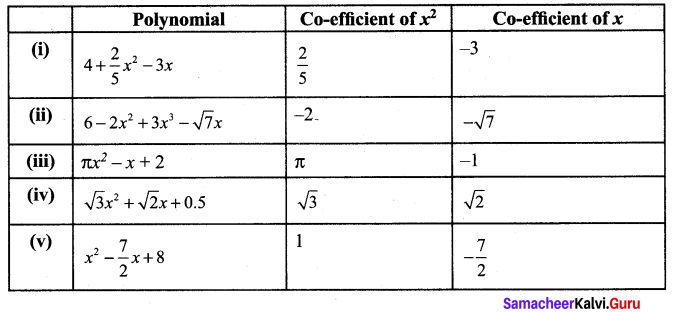9th Maths Exercise 3.1 Solutions Question 3.
Find the degree of the following polynomials.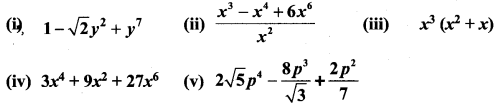Solution: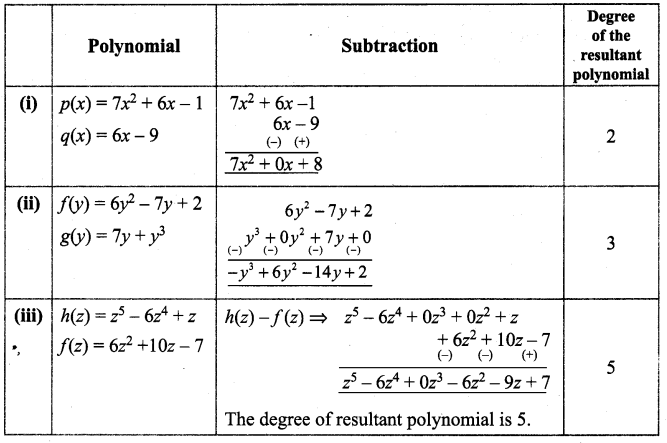Samacheer Kalvi Guru 9th Maths Question 4.
Rewrite the following polynomial in standard form.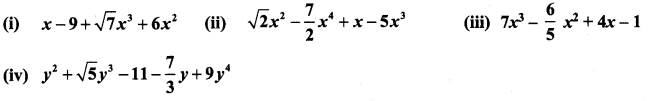Solution: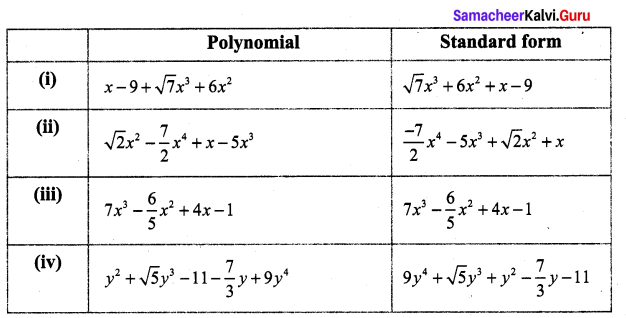9th Standard Maths Algebra Question 5.
Add the following polynomials and find the degree of the resultant polynomial.
(i) p(x) = 6x2 – 7x+ 2 q(x) = 6x3 – 7x + 15
(ii) h(x) = 7x3 – 6x + 1 f(x) = 7x2 + 17x – 9
(iii) f(x) = 16x4 – 5x2 + 9 g(x) = -6x3 + 7x – 15
Solution: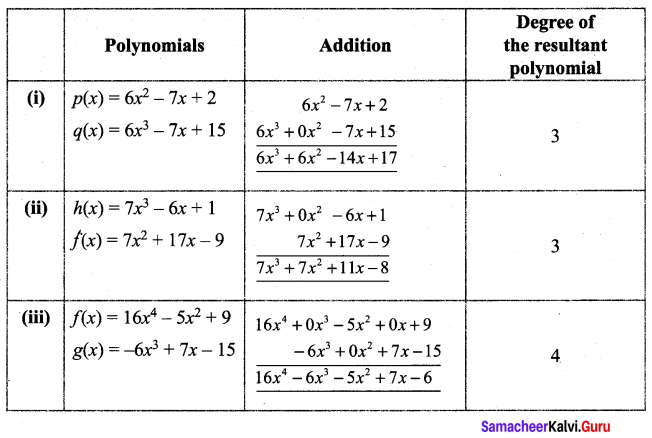9th Standard Maths Exercise 3.1 Question 6.
Subtract the second polynomial from the first polynomial and find the degree of the resultant polynomial.
(i) p(x) = 7x2 + 6x – 1 q(x) = 6x – 9
(ii) f(y) = 6y2 – 7y + 2 g(y) = 7y + y3
(iii) h(z) = z5 – 6z4 + z f(z) = 6z2 + 10z – 7
Solution:Samacheer Kalvi Guru 9 Maths Question 7.
What should be added to 2x3 + 6x2 – 5x + 8 to get 3x3 – 2x2 + 6x + 15?
Solution:
(2x3 + 6x2 – 5x + 8) + Q(x) = 3x3 – 2x2 + 6x + 15
∴ Q(x) = (3x3 – 2x2 + 6x + 15) – (2x3 + 6x2 – 5x + 8)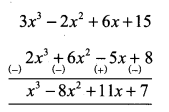The required polynomial is x3 – 8x2 + 11x + 7

Algebra 9th Standard Question 8.
What must be subtracted from 2x24 + 4x2 – 3x + 7 to get 3x3 – x2 + 2x + 1 ?
Solution:
(2x4 + 4x2 – 3x + 7) – Q(x) = 3x3 – x2 + 2x + 1
Q(x) = (2x4 + 4x2 – 3x + 7) – 3x3 – x2 + 2x + 1
The required polynomial = 2x4 + 4x2 – 3x + 7 – 3x3 + x2 – 2x – 1
= 2x4 – 3x3 + 5x2 – 5x + 6

Ex 3.1 Class 9th Maths Solution Question 9.
Multiply the following polynomials and find the degree of the resultant polynomial:
(i) p(x) = x2 – 9 q(x) = 6x2 + 7x – 2
(ii) f(x) = 7x + 2 g(x) = 15x – 9
(iii) h(x) = 6x2 – 7x + 1 f(x) = 5x – 7
Solution:
(i) p(x) = x2 – 9 q(x) = 6x2 + 7x – 2
p(x) × q(x) = (x2 – 9) (6x2 + 7x – 2)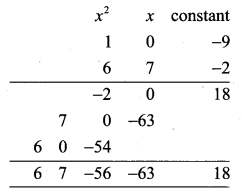The required polynomial is 6x4 + 7x3 – 56x2 – 63x + 18, degree 4.

(ii) f(x) = 7x + 2 g(x) = 15x – 9
f(x) × g(x) = (7x + 2) (15x – 9)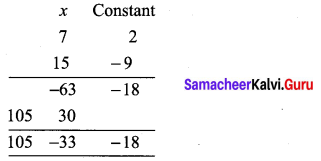The required polynomial is 105x2 – 33x – 18, degree 2.

(iii) h(x) = 6x2 – 7x + 1 f(x) = 5x – 7
h(x) × f(x) = (6x2 – 7x + 1) (5x – 7)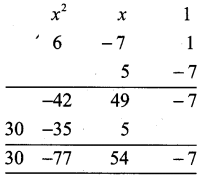The required polynomial is 30x3 – 77x2 + 54x – 7, degree 3.

9th Class Math Exercise 3.1 Solution Question 10.
The cost of chocolate is Rs. (x + y) and Amir bought (x + y) chocolates. Find the total amount paid by him in terms of x andy. If x = 10, y = 5 find the amount paid by him.
Solution:
Amount paid = Number of chocolates × Cost of a chocolate
= (x + y) (x + y) = (x + y)2 = x2 + 2xy + y2
If x = 10, y = 5
The total amount paid by him
= 102 + 2 × 10 × 5 + 52 = 100 + 100 + 25 = Rs. 225

Samacheer Kalvi Guru 9th Maths Guide Question 11.
The length of a rectangle is (3x + 2) units and it’s breadth is (3x – 2) units. Find its area in terms of x. What will be the area if x = 20 units.
Solution:
Area of a rectangle = length × breadth
= (3x + 2) × (3x – 2) = (3x)2 – 22 = [9x2 – 4] Sq. units
If x = 20, Area = 9 × 202 – 4 = 9 × 400 – 4
= 3600 – 4 = 3596 Sq. units

Samacheer Guru 9th Maths Question 12.
p(x) is a polynomial of degree 1 and q(x) is a polynomial of degree 2. What kind of the polynomial p(x) × q(x) is ?
Solution:
p(x) is a polynomial of degree 1. q(x) is a polynomial of degree 2.
Then the p(x) × q(x) will be the polynomial of degree (1 + 2) = 3 (or)
Cubic polynomial